# EML 3004 C CHAPTER 8 Dimensions Units and

• Slides: 12
Download presentationEML 3004 C CHAPTER 8 Dimensions, Units, and Error Namas Chandra Introduction to Mechanical engineering Chapter 8 -1EML 3004 C Objectives Understand the difference between a unit and dimensions Convert quantities between the different systems of measurement Understand the types of error in a measurement Express large numbers in scientific notation Sections 8. 1 8. 2 8. 3 8. 4 8. 5 8. 6 Introduction Dimensions and Units Conversion between Different Units Errors and Accuracy Significant Digits Summary Namas Chandra Introduction to Mechanical engineering Chapter 8 -2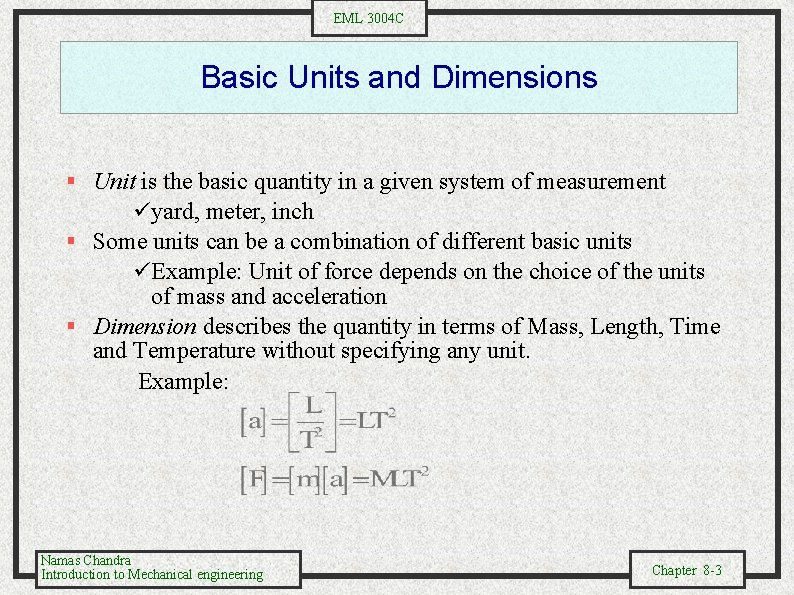EML 3004 C Basic Units and Dimensions § Unit is the basic quantity in a given system of measurement üyard, meter, inch § Some units can be a combination of different basic units üExample: Unit of force depends on the choice of the units of mass and acceleration § Dimension describes the quantity in terms of Mass, Length, Time and Temperature without specifying any unit. Example: Namas Chandra Introduction to Mechanical engineering Chapter 8 -3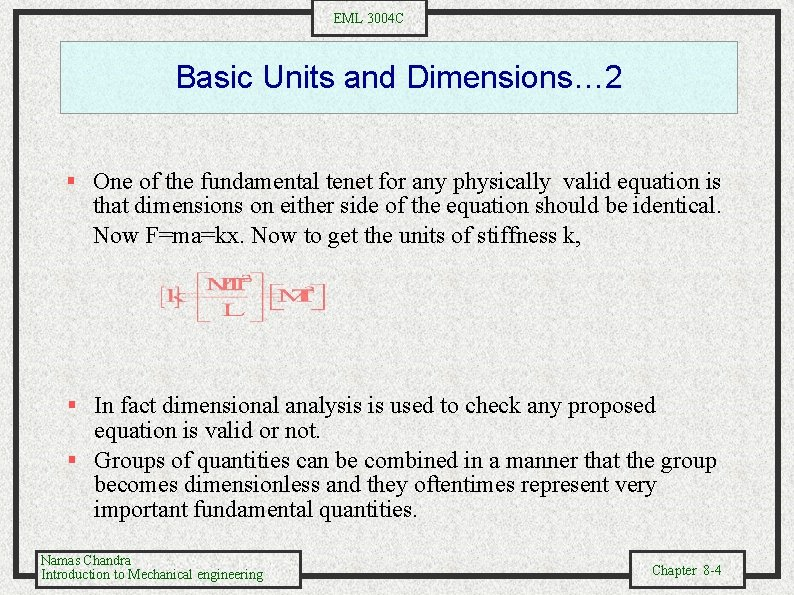EML 3004 C Basic Units and Dimensions… 2 § One of the fundamental tenet for any physically valid equation is that dimensions on either side of the equation should be identical. Now F=ma=kx. Now to get the units of stiffness k, § In fact dimensional analysis is used to check any proposed equation is valid or not. § Groups of quantities can be combined in a manner that the group becomes dimensionless and they oftentimes represent very important fundamental quantities. Namas Chandra Introduction to Mechanical engineering Chapter 8 -4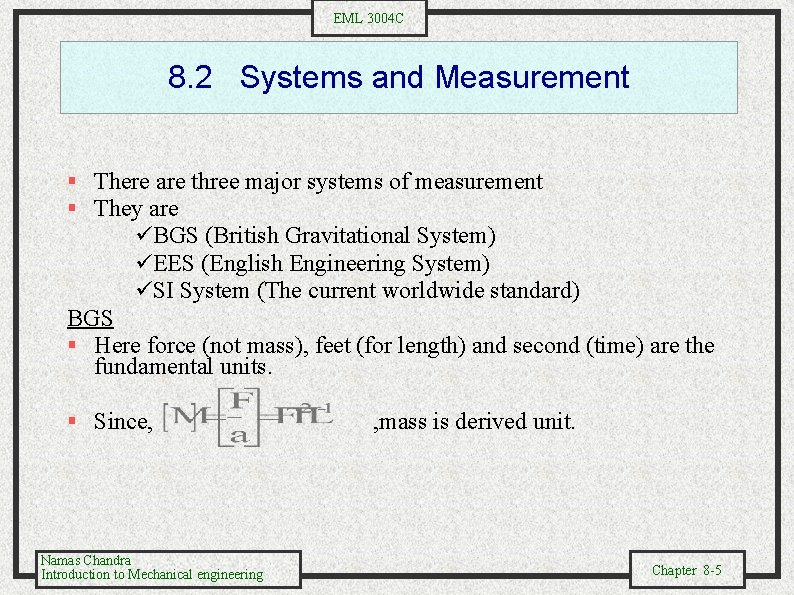EML 3004 C 8. 2 Systems and Measurement § There are three major systems of measurement § They are üBGS (British Gravitational System) üEES (English Engineering System) üSI System (The current worldwide standard) BGS § Here force (not mass), feet (for length) and second (time) are the fundamental units. § Since, Namas Chandra Introduction to Mechanical engineering , mass is derived unit. Chapter 8 -5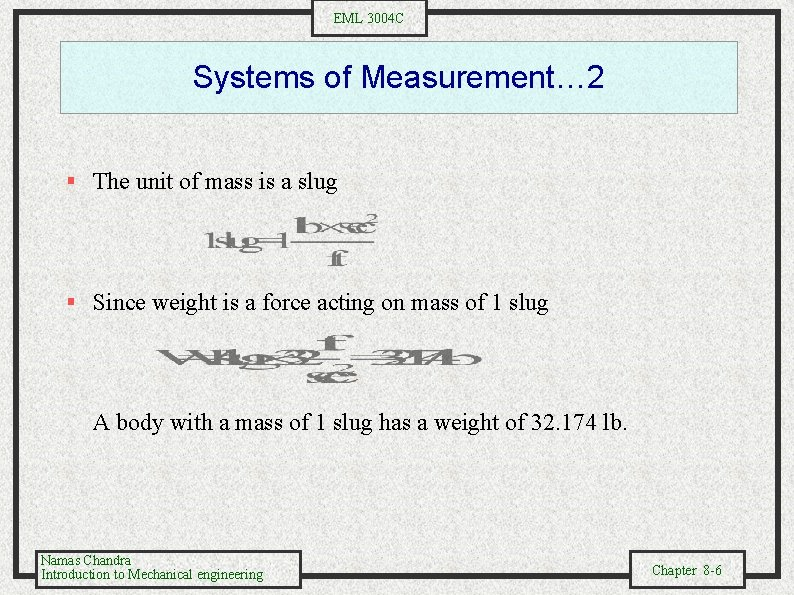EML 3004 C Systems of Measurement… 2 § The unit of mass is a slug § Since weight is a force acting on mass of 1 slug A body with a mass of 1 slug has a weight of 32. 174 lb. Namas Chandra Introduction to Mechanical engineering Chapter 8 -6EML 3004 C Systems and Measurement… 3 EES § Here the unit of mass is defined such that weight is 1 lbf § Mass is 1 lbm § For this system use SI § SI is the metric system. Fundamental units are grams (weight), cm ( length), and seconds (time). Namas Chandra Introduction to Mechanical engineering Chapter 8 -7EML 3004 C Conversion between Units § We are faced with converting a quantity from one system to another. Use the logic that units should cancel on both sides Example: Conversion of FPS Force 1 lb Mass 1 slug Length 1 ft Namas Chandra Introduction to Mechanical engineering = = = SI 4. 4482 N 14. 5938 kg 0. 3048 m Chapter 8 -8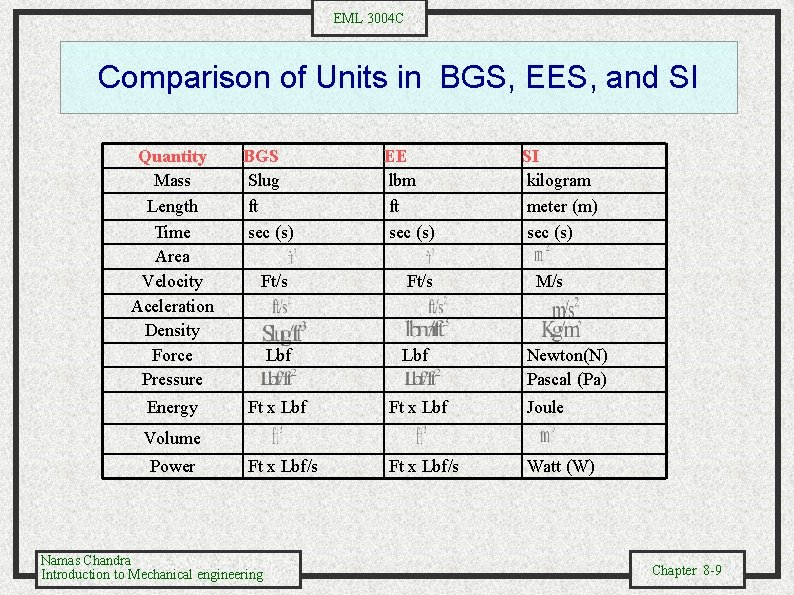EML 3004 C Comparison of Units in BGS, EES, and SI Quantity Mass Length Time Area Velocity Aceleration Density Force Pressure Energy BGS Slug ft sec (s) EE lbm ft sec (s) SI kilogram meter (m) sec (s) Ft/s M/s Lbf Ft x Lbf Joule Ft x Lbf/s Watt (W) Newton(N) Pascal (Pa) Volume Power Namas Chandra Introduction to Mechanical engineering Chapter 8 -9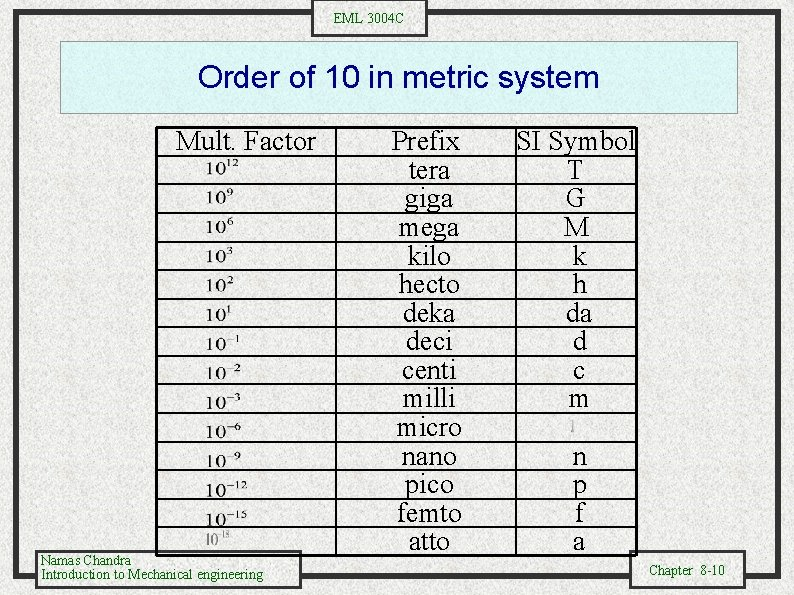EML 3004 C Order of 10 in metric system Mult. Factor Namas Chandra Introduction to Mechanical engineering Prefix tera giga mega kilo hecto deka deci centi milli micro nano pico femto atto SI Symbol T G M k h da d c m n p f a Chapter 8 -10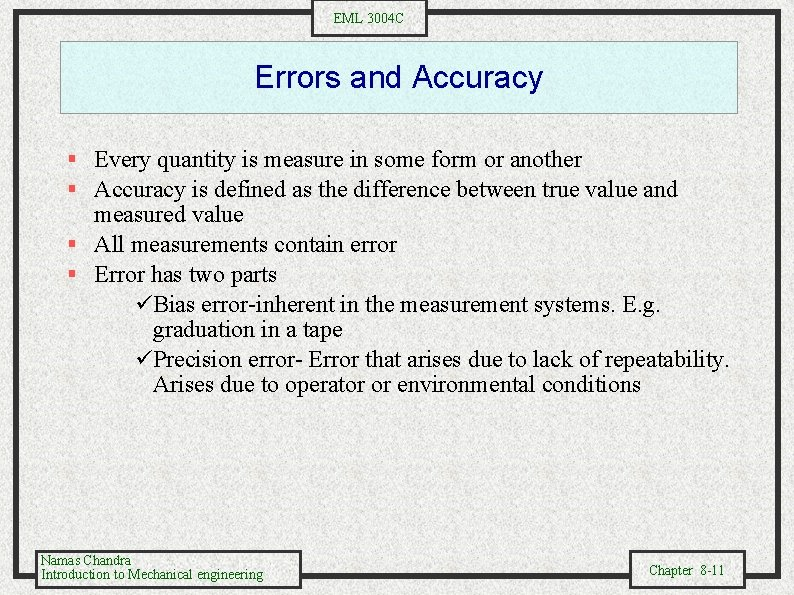EML 3004 C Errors and Accuracy § Every quantity is measure in some form or another § Accuracy is defined as the difference between true value and measured value § All measurements contain error § Error has two parts üBias error-inherent in the measurement systems. E. g. graduation in a tape üPrecision error- Error that arises due to lack of repeatability. Arises due to operator or environmental conditions Namas Chandra Introduction to Mechanical engineering Chapter 8 -11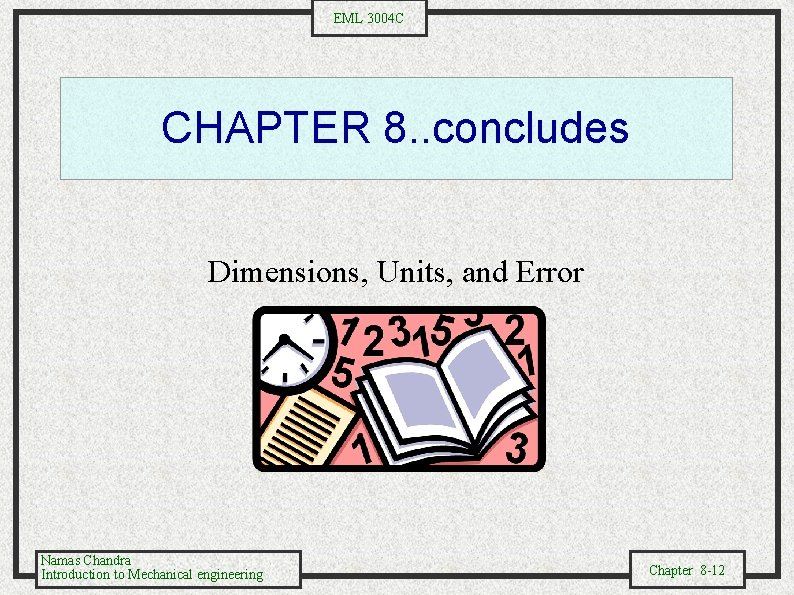EML 3004 C CHAPTER 8. . concludes Dimensions, Units, and Error Namas Chandra Introduction to Mechanical engineering Chapter 8 -12